# Standard deviation (std)

In probability and statistics, the standard deviation of a random variable is the average distance of a random variable from the mean value.

It represents how the random variable is distributed near the mean value. Small standard deviation indicates that the random variable is distributed near the mean value. Big standard deviation indicates that the random variable is distributed far from the mean value.

### Standard deviation definition formula

The standard deviation is the square root of the variance of random variable X, with mean value of μ.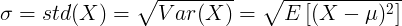From the definition of the standard deviation we can get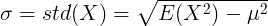### Standard deviation of continuous random variable

For continuous random variable with mean value μ and probability density function f(x):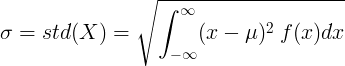or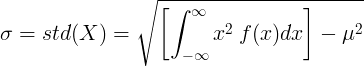### Standard deviation of discrete random variable

For discrete random variable X with mean value μ and probability mass function P(x):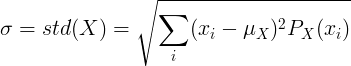or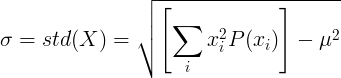Probability distribution »

Currently, we have around 929 calculators and conversion tables to help you "do the math" quickly in areas such as:

And we are still developing more. Our goal is to become the one-stop, go-to site for people who need to make quick calculations or who need to find quick answer for basic conversions.

Additionally, we believe the internet should be a source of free information. Therefore, all of our tools and services are completely free, with no registration required. We coded and developed each calculator individually and put each one through strict, comprehensive testing. However, please inform us if you notice even the slightest error – your input is extremely valuable to us. While most calculators on Justfreetools.com are designed to be universally applicable for worldwide usage, some are for specific countries only.